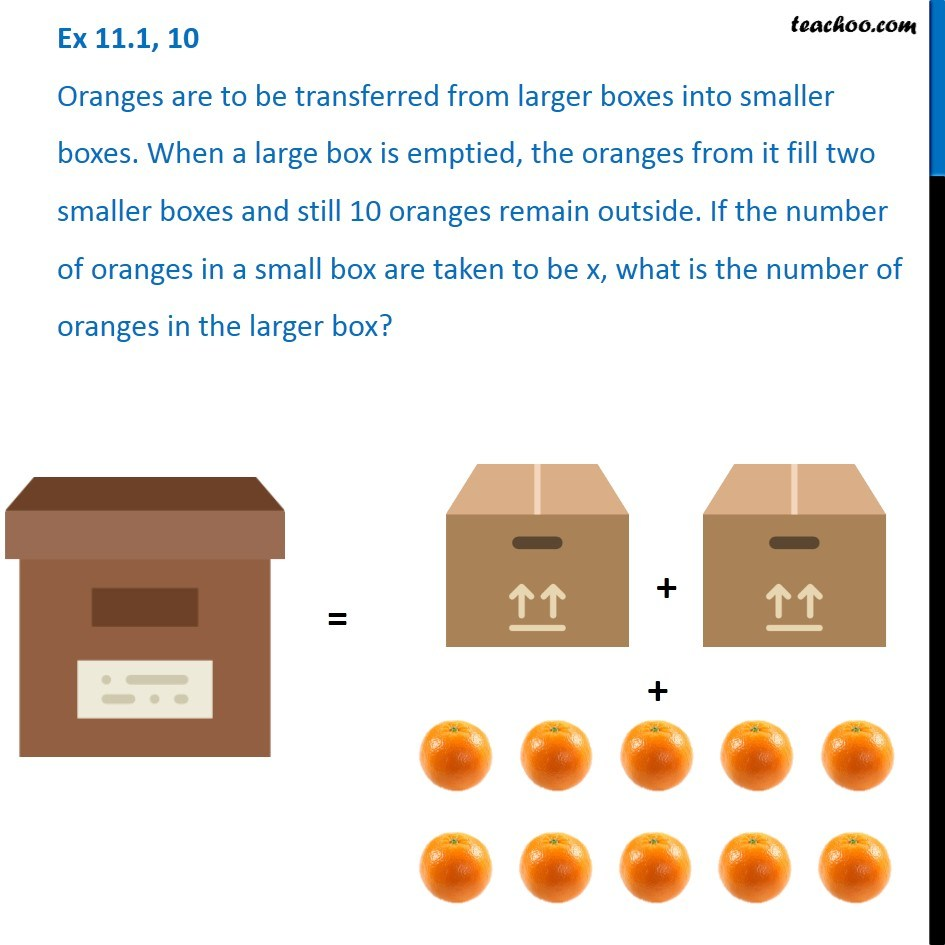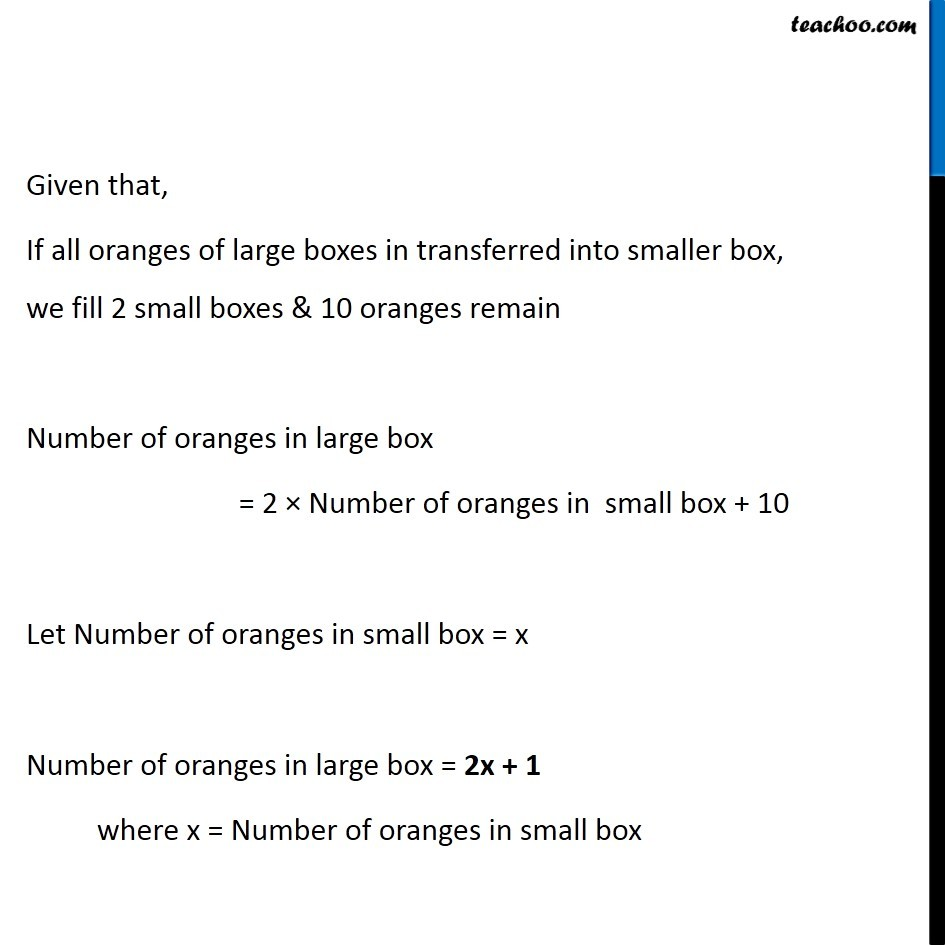1. Chapter 11 Class 6 Algebra
2. Concept wise
3. Variable

Transcript

Ex 11.1, 10 Oranges are to be transferred from larger boxes into smaller boxes. When a large box is emptied, the oranges from it fill two smaller boxes and still 10 oranges remain outside. If the number of oranges in a small box are taken to be x, what is the number of oranges in the larger box? Given that, If all oranges of large boxes in transferred into smaller box, we fill 2 small boxes & 10 oranges remain Number of oranges in large box = 2 × Number of oranges in small box + 10 Let Number of oranges in small box = x Number of oranges in large box = 2x + 1 where x = Number of oranges in small box

Variable